# Connected space

(Redirected from Connectedness)
Jump to: navigation, search

## Definition

### Equivalent definitions in tabular format

A topological space is said to be connected if it satisfies the following equivalent conditions.

No. Shorthand A topological space is termed connected if ... A topological space$X$ is termed connected if ...
1 Absence of two open subset separation it cannot be expressed as a disjoint union of two nonempty open subsets. it is not possible to find open subsets$U, V \subseteq X$ such that$U, V$ are both nonempty,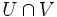$U \cap V$ is empty, and$U \cup V = X$.
2 Absence of two closed subset separation it cannot be expressed as a disjoint union of two nonempty closed subsets. it is not possible to find closed subsets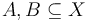$A, B \subseteq X$ such that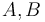$A, B$ are both nonempty,$A \cap B$ is empty, and$A \cup B = X$.
3 Absence of open subset separation it cannot be expressed as a union of a collection of pairwise disjoint nonempty open subsets that has size more than one. it is not possible to write$X$ as a union$\bigcup_{i \in I} U_i$ where$U_i$ are all nonempty and open,$U_i \cap U_j$ is empty for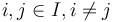$i, j \in I, i \ne j$, and$I$ has size greater than one.
4 Absence of finite closed subset separation it cannot be expressed as a union of a collection of finitely many pairwise disjoint nonempty closed subsets that has size more than one. it is not possible to write$X$ as a union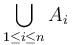$\bigcup_{1 \le i \le n} A_i$ where$A_i$ are all nonempty and closed,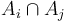$A_i \cap A_j$ is empty for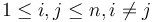$1 \le i,j \le n, i \ne j$, and$n \ge 1$.
5 Absence of proper nonempty clopen subset the only clopen subsets of the space are the whole space and the empty subset. for any subset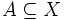$A \subseteq X$ such that$A$ is clopen, i.e.,$A$ is both open and closed, either$A$ is empty or$A = X$.

The term is typically used for non-empty topological spaces. Whether the empty space can be considered connected is a moot point.

## Metaproperties

Metaproperty name Satisfied? Proof Statement with symbols
subspace-hereditary property of topological spaces No connectedness is not hereditary It is possible to have a connected space$X$ and a subset$A$ of$X$ such that$A$ is not connected in the subspace topology.
weakly hereditary property of topological spaces No connectedness is not weakly hereditary It is possible to have a connected space$X$ and a closed subset$A$ of$X$ such that$A$ is not connected in the subspace topology.
product-closed property of topological spaces Yes connectedness is product-closed Suppose$X_i, i \in I$, are all connected spaces. Then, the Cartesian product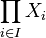$\prod_{i \in I} X_i$ is also a connected space with the product topology.
box product-closed property of topological spaces No connectedness is not box product-closed It is possible to have$X_i, i \in I$ all connected spaces such that the Cartesian product$\prod_{i \in I} X_i$ is not connected in the box topology.
coarsening-preserved property of topological spaces Yes connectedness is coarsening-preserved If$X$ is connected under a topology$\tau$, it remains connected when we pass to a coarser topology than$\tau$.
continuous image-closed property of topological spaces Yes connectedness is continuous image-closed If$X$ is a connected space and$Y$ is the image of$X$ under a continuous map, then$Y$ is also connected.
connected union-closed property of topological spaces Yes connectedness is connected union-closed
closure-preserved property of topological spaces Yes connectedness is closure-preserved Suppose$A$ is a subset of$X$ that is connected in the subspace topology. Then, the closure$\overline{A}$ is also connected in its subspace topology.

## Facts

Any topological space (not necessarily connected) can be partitioned into its connected components. The space is connected iff it has a single connected component, namely the whole space itself.

This article defines a homotopy-invariant property of topological spaces, i.e. a property of homotopy classes of topological spaces

View other homotopy-invariant properties of topological spaces OR view all properties of topological spaces

This article defines a property of topological space that is pivotal (viz important) among currently studied properties of topological spaces

This article is about a basic definition in topology.
VIEW: Definitions built on this | Facts about this | Survey articles about this
View a complete list of basic definitions in topology

## Relation with other properties

### Stronger properties

Property Meaning Proof of implication Proof of strictness (reverse implication failure) Intermediate notions
path-connected space path joining any two points path-connected implies connected connected not implies path-connected |FULL LIST, MORE INFO
simply connected space path-connected, trivial fundamental group |FULL LIST, MORE INFO
contractible space homotopy-equivalent to a point |FULL LIST, MORE INFO
irreducible space |FULL LIST, MORE INFO
ultraconnected space |FULL LIST, MORE INFO

### Weaker properties

Property Meaning Proof of implication Proof of strictness (reverse implication failure) Intermediate notions
space with finitely many connected components |FULL LIST, MORE INFO
space with finitely many quasicomponents Space with finitely many connected components|FULL LIST, MORE INFO
space in which all connected components are open Space with finitely many connected components|FULL LIST, MORE INFO
space in which the connected components coincide with the quasicomponents Space in which all connected components are open|FULL LIST, MORE INFO

### Relation with size of space

Combining connectedness with a separation axiom usually yields a lower bound on the cardinality of the space as long as it has at least two points. Below are some examples of such facts:

Other property What its combination with being connected gives us if it has at least two points Proof
T1 space infinite space. In fact, any finite T1 space must be discrete connected and T1 with at least two points implies infinite
regular Hausdorff space uncountable space connected and regular with at least two points implies uncountable
Urysohn space uncountable space, cardinality at least that of the continuum connected and Urysohn with at least two points implies cardinality at least that of the continuum
normal Hausdorff space uncountable space, cardinality at least that of the continuum connected and normal Hausdorff with at least two points implies cardinality at least that of the continuum